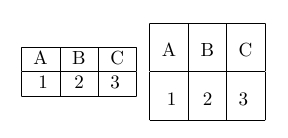chaning row height

# LaTeX row height

StartLaTeX faqLaTeX topicsLaTeX course
< table landscape     > centering a table

## row height

How to change row height of tables in LaTeX.

### Problem

The height of cell could be not high enough for some reasons.

### Solution

If you just want to change height of all rows, just wrote \renewcommand{\arraystretch}{parameter} before this table.

### Example

\documentclass{article}
\begin{document}

\begin{tabular}{|r|c|l|}
\hline
A & B & C \\
\hline
1 & 2 & 3 \\
\hline
\end{tabular}
\renewcommand{\arraystretch}{2}
\begin{tabular}{|r|c|l|}
\hline
A & B & C \\
\hline
1 & 2 & 3 \\
\hline
\end{tabular}
\renewcommand{\arraystretch}{1}
\end{document}

Output:### Hint

After using this renewcommand, you should change the size back to 1 by \renewcommand{\arraystretch}{1}. If you do not change it back every following array and table would become an icrease in height.

### changing one row

To change only one particular row you could use the command \rule with a width of zero and the height you want as parameter, for example \rule{0pt}{25pt}

### example 2

\documentclass{article}
\begin{document}

\begin{tabular}{|r|c|l|}
\hline
A & B & C \\
\hline
1 & 2 & 3 \\
\hline
\end{tabular}
\begin{tabular}{|r|c|l|}
\hline
A & B & C \\
\hline
\rule{0pt}{25pt}1 & 2 & 3 \\
\hline
\end{tabular}
\end{document}

Output:Contact